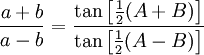# Triangle Identities

## Triangle Identities

The triangle identities are equations that are true for all triangles (they don't need to have a right angle). For the identities involving right angles triangles see Trigonometric Identities.### Law of Sines

The Law of Sines (also known as The Sine Rule) is: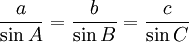it can also be this way around: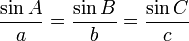### Law of Cosines

The Law of Cosines (also known as The Cosine Rule) is an extension of the Pythagorean Theorem to any triangle: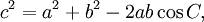which can also be re-arranged to: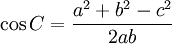### Law of Tangents

The Law of Tangents is: